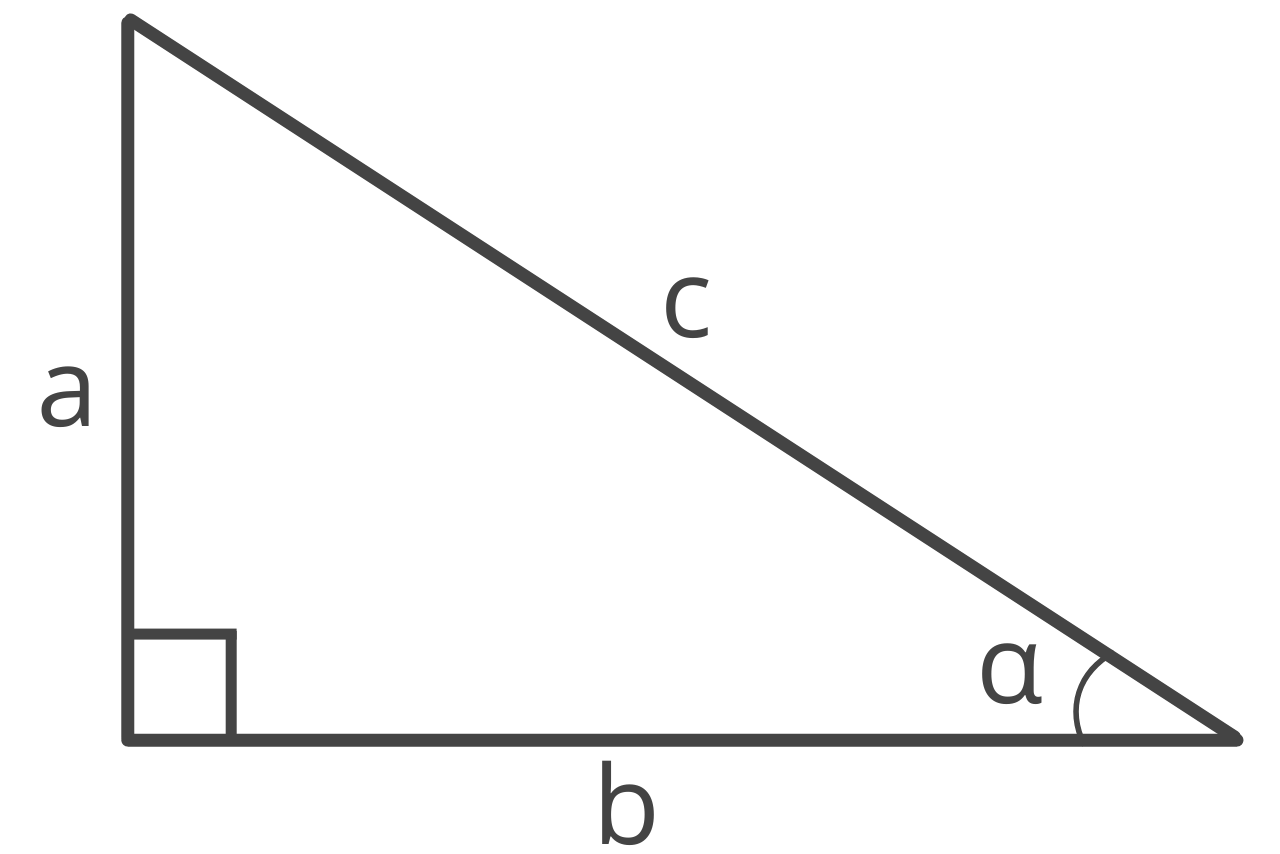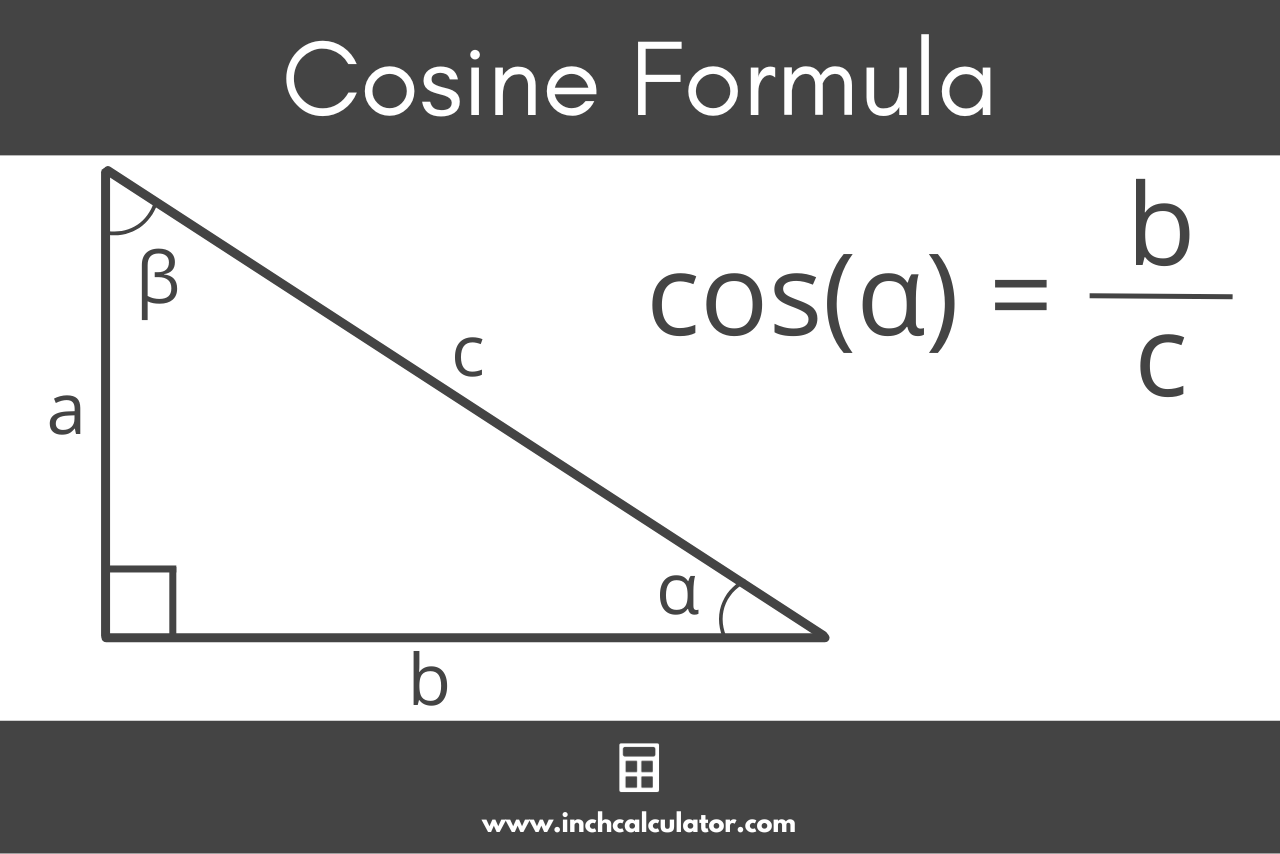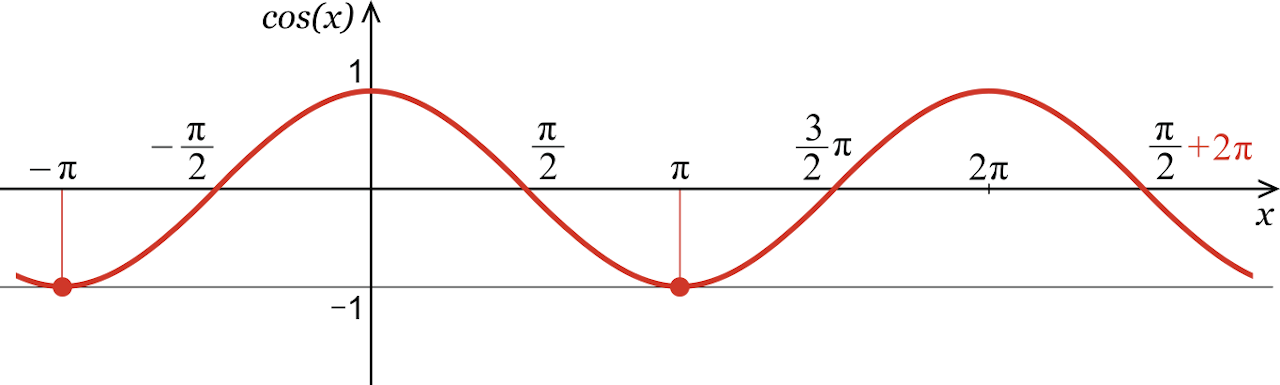# Cosine Calculator – Calculate cos(x)

Find the cosine of an angle using the cos calculator below. Start by entering the angle in degrees or radians.

## How to Find the Cosine of an Angle

In a right triangle, the cosine of angle α, or cos(α), is the ratio between the adjacent side of an angle and the hypotenuse.Cosine is one of the three primary trigonometric functions and is abbreviated cos.

You might be curious how to find the cosine of an angle. Use the formula below to calculate cos.

### Cosine Formula

The cosine formula is:

cos(α) = adjacent / hypotenuse = b / c

Thus, the cosine of angle α in a right triangle is equal to the adjacent side’s length divided by the hypotenuse.

To find the ratio of cosine, simply enter the length of the adjacent and hypotenuse and simplify.For example, let’s calculate the cosine of angle α in a triangle with the length of the adjacent side equal to 6 and the hypotenuse equal to 8.

cos(α) = 6 / 8
cos(α) = 3 / 4

## Cosine Graph

If you graph the cosine function for every possible angle, it forms a repeating up/down curve. This is known as a cosine wave.The curve begins at the maximum, (0, 1), because cos(0) = 1. As cosine approaches π/2, the value decreases to the x-axis. The value then continues to decrease to the minimum value of -1 at π. The function increases to the x-axis at 3π/2 and completes its period at 2π as it returns to the maximum of 1.

The cosine function continues indefinitely and has a period of 2π. The maximum and minimum values occur in each period and are separated by a distance of one period.

Thus, the key points on a cosine graph are (0, 1), (π/2, 0), (π, -1), (3π/2, 0), and (2π, 1).

## Cosine Table

The table below shows common angles and the cos value for each of them.

Table showing common angles and cosine values for each.
0 1
15° π / 12 √6 + √2 / 4
30° π / 6 √3 / 2
45° π / 4 √2 / 2
60° π / 3 1 / 2
75° / 12 √6 – √2 / 4
90° π / 2 0
105° / 12 √6 – √2 / 4
120° / 3 1 / 2
135° / 4 √2 / 2
150° / 6 √3 / 2
165° 11π / 12 √6 + √2 / 4
180° π -1
195° 13π / 12 √6 + √2 / 4
210° / 6 √3 / 2
225° / 4 √2 / 2
240° / 3 1 / 2
255° 17π / 12 √6 – √2 / 4
270° / 2 0
285° 19π / 12 √6 – √2 / 4
300° / 3 1 / 2
315° / 4 √2 / 2
330° 11π / 6 √3 / 2
345° 23π / 12 √6 + √2 / 4
360° 1

## Inverse Cosine

The inverse of the cosine function is the arccos function. Thus, if you know the ratio of cosine of an angle, you can use arccos to find the measurement of the angle.

Arccos can also be expressed as cos-1(x)

## Secant

Secant, on the other hand, is a separate trigonometric function that is the reciprocal of the cosine value. The following formulas show the relationship between cosine and secant.

cos(α) = adjacent / hypotenuse = b / c
sec(α) = hypotenuse / adjacent = c / b = 1 / cos(α)

Note that secant is not the same as arccos.

You might also be interested in our sine and tangent calculators.

## Law of Cosines

The Law of Cosines connects a triangle’s side lengths and angles, making it important for solving non-right triangles where the Pythagorean theorem cannot be used.

Stated as c² = a² + b² – 2ab * cos(θ) for a triangle with sides a, b, and c and angle θ opposite side c, the Law of Cosines is used to find an unknown side length given two sides and the included angle, or to calculate an angle when all side lengths are known.

### Do you use degrees or radians with cosine?

You can use both degrees and radians with cosine, but it is important to know which one you are using since each produces different results. Radians are more commonly used in mathematics and programming languages. To use degrees, you must convert them to radians using the formula:

radians = (degrees × π) / 180

Our degrees to radians calculator is also an easy way to do this conversion.

### Can you find cosine without a calculator?

You can find approximate cosine values without a calculator by using the cosine ratio. Cosine A is equal to the length of the leg adjacent to angle A divided by the length of the hypotenuse.

### How do you convert cosine to an angle?

To convert a cosine value to an angle, you need to find the inverse of the cosine function, called the arccosine function (cos-1 or acos). The arccosine function takes the cosine value as input and returns the corresponding angle.

Calculators with built-in arccosine functions can be used to find the angle, which is typically given in radians. To convert the result to degrees, use the formula:

degrees = (radians × 180) / π

You can use our radians to degrees calculator to convert an angle to degrees as well.

### Does cosine repeat?

The cosine function is periodic with a period of 2π radians (or 360° in degrees), meaning its values repeat at regular intervals due to the unit circle’s periodic nature.